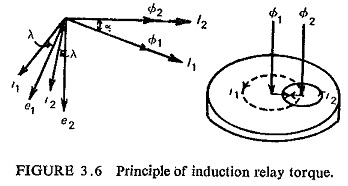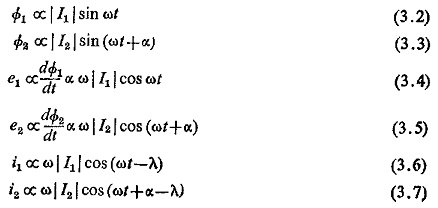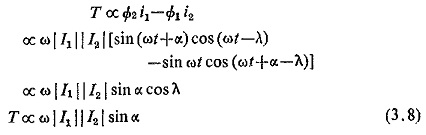## Induction Relay Torque Equation:

In Induction Relay Torque Equation, Two magnetic fluxes Φ1 and Φ2 differing in time phase penetrate through a disc. These alternating fluxes induce emfs e1 and e2 in the disc which lag their respective fluxes by 90°. These emfs lead to the flow of eddy currents i1 and i2. By the interaction of Φ1 with i2 and Φ2 with i1 a driving torque is produced.

The currents i1 and i2 lag the voltages e1 and e2 by the impedance angle λ of the disc. Figure (3.6) shows the vector diagram.LetTherefore, the resultant torque is given by.Thus the induction relay is a sine comparator in which the maximum torque is developed when α is 90° or 270° and zero torque when α is 0° or 180°.

Scroll to Top